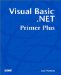# Review Questions

When you answer the questions that involve writing code, you can place the statements in the form Load event and place a breakpoint at the End Sub statement at the end of the form Load event. You can then place the cursor over each variable you use and it will show you the value.

 1: What is a string variable? A1: A string variable is used to store text data in memory. 2: What is the difference between a string variable and a string literal? A2: A string variable can hold any sequence of character data that might be assigned to it. A string literal is a sequence of characters that are surrounded by quotation marks. In the statement ` buff = "This is a string literal" ` the variable buff is a string variable and the phrase surrounded by quotes is a string literal. 3: Suppose you want to extract the first name from a string. How would you do it? For example, if the name is Jennifer Hartman , how would you extract Jennifer from the string? A3: First, think about what you want to do. You need to separate the first and last names into two parts and retrieve the first part. You know they are separated by a blank space. Therefore, this problem can be solved using a two-step process: 1) Find where the blank space is, and 2) return everything to the left of the blank space. The code would be ` Dim Blank As Integer, buff As String, First As String buff = "Jennifer Hartman" Blank = InStr(buff, " ") First = Microsoft.VisualBasic.Left\$(buff, Blank - 1) ` The InStr() function returns the character position of where the blank space is. The Left\$() function returns the leftmost characters from the string named buff starting at position 0 and going to position Blank “1. If we didn't subtract the 1, we would also have the blank space as part of the first name. 4: With Option Strict turned on, Visual Basic .NET does not allow blind casts. (A blind cast is when you try to assign one data type into a different data type.) With that in mind, fix the following statement: ` txtResult.Text = 10 ` A4: You need to avoid a blind cast by using a type converter: ` TxtResult.Text = CStr(10) ` 5: What's the difference with respect to string between a shared method and an instance method? A5: A shared method is one that is inherent to the string class. For example, the statement ` buff = String.Concat(s1, s2, s3) ` is a shared method that is inherent for all string class variables . On the other hand, an instance method exists only for an instance of the string object. An example would be ` length = buff.Length ` where Length is an instance method that is associated with the buff string. 6: It's not uncommon to pack data into a string. Suppose that you want to keep information about club members . Let's further assume that the first two bytes are the number of years the person has been a member, the next byte is the member's classification (that is, a Junior, Regular, or Senior member), and the next four characters is the person's club number. Extract the information for the string: ` "15R0501" ` A6: You can use the substring instance method to derive the results: ` Dim Years As Integer, Type As String, Num As Integer buff = "15R0501" Years = CInt(buff.Substring(0, 2)) Type = buff.Substring(2, 1) Num = CInt(buff.Substring(3)) ` The Substring() method is overloaded. This means you can use it in more than one way. In the first two uses, the first argument is the starting position within the string, and the second argument is the number of characters you want to examine. In the last use, you simply pass in the starting position. In that instance, Substring uses everything from that position to the end of the string. 7: What is the difference between and ASCII and Unicode character sets? A7: An ASCII character only uses 7 bits to represent a character. As a result, ASCII defines 128 characters in the ASCII character set. If you look at your keyboard, you'll see that there are fewer than 128 characters shown, so ASCII works fine for the characters we use in the English character set. Unicode characters are 16-bit characters, which means that there can be more than 65,000 different characters in a Unicode character set. Some character sets, such as Chinese or Japanese, may use thousands of characters in their character sets. The Unicode character set accommodates their needs. By default, Visual Basic .NET uses the Unicode character set. 8: Suppose that you have a large block of text stored in a variable named buff . You want to search the text for the substring Vanessa to find its position in the text. How would you do this? A8: You would use the IndexOf() instance method: ` Position = buff.IndexOf("Vanessa") ` If the string is found, Position will hold the index value of where the substring starts. If Position is -1 , the substring was not found.Visual Basic .NET Primer Plus
ISBN: 0672324857
EAN: 2147483647
Year: 2003
Pages: 238
Authors: Jack Purdum

Similar book on Amazon